# Dynamic Response of a Partially Debonded Circular Lined Tunnel in the Overburden to SH-Wave

Dynamic Response of a Partially Debonded Circular Lined Tunnel in the Overburden to SH-Wave
College of Aerospace and Civil Engineering, Harbin Engineering University, Harbin 150001, China

Corresponding Author Email:
zhangyang1987@hrbeu.edu.cn
Page:
321-331
|
DOI:
https://doi.org/10.18280/ijsse.100303
17 February 2020
|
Accepted:
27 April 2020
|
Published:
30 June 2020
| Citation

OPEN ACCESS

Abstract:

Using complex variable function and wave function expansion, this paper obtains the analytical solution to the scattering of steady-state planar SH-wave on a partially debonded circular lined tunnel in the overburden, and analyzes the accuracy of the solution. By the big circle method, the straight boundary between soil layers was converted into an arc boundary. Under continuous boundary conditions, the scattered wave field of debonded structure was constructed through Fourier series expansion, eliminating the need to process singular points on the edge of the debonded lining. According to the boundary conditions, a set of infinite integral equations was established, and solved by intercepting finite terms. After that, a numerical example was introduced to reveal how the dynamic stress concentration factor (DSCF) of the lining is affected by incident frequency, soil layer parameters, lining material, and debonding position. The results show that the DSCF of the partially debonded circular lined tunnel was obviously amplified by the soft overburden, but shielded by the hard overburden; Judging by the distribution and value of outer wall DSCF, rigid lining is affected by debonding more significantly than flexible lining.

Keywords:

scattering of SH-wave, overburden, lining, big circle method, dynamic stress concentration factor (DSCF), analytical solution

1. Introduction

In the event of an earthquake, the dynamic stress will concentrate around tunnels, as the seismic wave is scattered repeatedly by the ground and the tunnels. Then, the local stress of the tunnel will bump up, causing damages to tunnel structure. Significant loss of life and property will occur, if the earthquake damages infrastructure like metro tunnels, heating pipelines, drainage pipelines, and oil pipelines. Therefore, the dynamic seismic response of underground structures has attracted much attention from the academia [1, 2].

Wave function expansion has been widely adopted to solve the response to seismic waves, after it was coupled by Baron with integral transform to obtain the analytical solution to how cylindrical cavity scatters compressive pulses [3, 4]. Liu et al. [5, 6] introduced complex variable function to the scattering problem of two-dimensional (2D) elastic dynamics, and solved the scattering of SH-wave by a shallow half space structure, using wave function expansion, complex variable function, and moving coordinate system. Later, Davis et al.  and Liang et al.  studied the scattering of planar P-wave and SV-wave by half space caves and arc-shaped depressions. Qi, Yang and Qi  and Qi et al.  extended the complex variable function to half space, half space interface, as well as circular cavities and inclusions in half space bidirectional medium, and explored the scattering of SH wave by circular lining near semi-infinite half space.

Under the effects of multiple factors (e.g. construction techniques, soil settlement, and changing groundwater level), the interface between a structure and the surrounding soil might separate in local places, forming debonded areas. Debonding can be regarded as a kind of cracking that separates the contact surfaces. In the 1980s, Coussy  studied the debonding between cylindrical inclusions and substrate in one place. In the 1990s, Yang and Norris [12-13] investigated the situation of a single arc crack, and provided the near-field and far-field solutions at arbitrary wavelengths. In the 21st century, Fang et al.  and Coşkun and Dolmaseven  explored intensively into the scattering problem of lined tunnels, yielding fruitful results with engineering significance.

The above studies mostly simplify the ground into a whole elastic half space. However, there is little report on the scattering by lined tunnel in the overburden. In reality, the ground is not a homogenous whole, but a mixture of various rocks or soil layers. The composite soil layer complicates the boundary conditions of the scattering problem, making the problem difficult to solve. At the end of the 20th century, Cao and Lee [16, 17] and Lee and Karl  transformed the traditional straight boundary problem into a curved boundary problem by the big circle method, and gave the analytical solutions to the scattering of P- and SV-waves by a single circular cavity in half space. Since then, the big circle method has been extensively implemented to study elastic waves [19-21]. With the aid of the big circle method, this paper aims to further expand the scattering problem of half space lining, under the effect of composite soil layer.

By the big circle method, this paper converts the straight boundary between two soil layers into an arc boundary, and constructs the scattered wave field of each soil layer. Then, the scattering problem of the model of the overburden was divided into the circular cavity scattering in the overburden (Domain II) and lining scattering by the circular tunnel (Domain III). Displacement solutions were constructed in Domains II and III, respectively, and fitted on the common boundaries between the two domains. Under continuous boundary conditions, the scattered wave field of debonded structure was constructed through Fourier series expansion, creating the analytical solution to the scattering problem. After that, the terms to be intercepted were identified by checking residual stress, which guarantee the accuracy of the numerical calculation. Taking C30 concrete and Q345 steel as lining materials, the SH-wave was incident to two typical geological combinations. On this basis, the authors analyzed how the dynamic stress concentration factor (DSCF) of the outer wall of the lining is affected by incident frequency, soil layer parameters, lining material, and debonding position.

2. Model Construction and Solution

The 2D model of the overburden containing a single circular lined tunnel is illustrated in Figure 1, where Domain I is the lower soil layer, Domain II is the upper overburden, and Domain III is the circular lined tunnel (inner diameter a; outer diameter b). For Domains I-III, the densities are ρ1, ρ2, and ρ3, the shear moduli are μ1, μ2, and μ3, the shear wave velocities are c1, c2, and c3, and the SH-wave velocities are k1, k2, and k3, respectively.

## 1.pngFigure 1. The 2D model of the overburden containing a single circular lined tunnel

Displacement solutions were constructed in Domains II and III, respectively, and fitted on the common boundaries between the two domains: stress and displacement should be continuous on the outer boundary T1 of the tunnel, and the stress should be free on the inner boundary T2 of the tunnel.

The upper boundary, lower boundary, and thickness of the overburden are denoted as TU, TD, and h, respectively. The distances from TU and TD to the center of the circular tunnel are denoted as h1 and h2, respectively. By the big circle method, TU and TD were approximated by concentric arcs with extremely large radii. Then, TU and TD were converted into $\widehat{T_{U}}$ and $\widehat{T_{D}}$, respectively.

Then, a rectangular coordinate system X1O1Y1 was established with the center of the big arc as the origin O1; another rectangular coordinate system X2O2Y2 was established with the center of the lined tunnel as the origin O2 and the straight line parallel to TU as axis X2, such that axes Y1 and Y2 are collinear.

The polar angle of any point in space can be expressed as θ1 under X1O1Y1, while that of any point on tunnel boundaries can be expressed as θ2 under X2O2Y2. If the outer wall of the lining is debonded, the starting and ending angles of the debonded structure are denoted as θ3 and θ4, respectively (θ4θ3). The debonded boundary and non-deboned boundary of the outer wall can be described as $\overline{T_{1}}$ and T1, respectively.

Suppose a steady-state SH-wave is incident from Domain I at the angle of α0. Then, the complex planes $\left(z_{1}, \bar{z}_{1}\right)$ and $\left(Z_{2}, \bar{Z}_{2}\right)$ that correspond to X1O1Y1 and X2O2Y2 can be established. On this basis, the relationships between the geometric variables can be depicted as:

$h=h_{1}+h_{2}, R_{U}=h+R_{D}, z_{2}=z_{1}-i\left(R_{D}+h_{2}\right)$     (1)

On plane $\left(z_{1}, \bar{z}_{1}\right),$ the displacement field and corresponding stresses of the incident wave $W^{(\mathrm{i})}$ in Domain I can be respectively expressed as :

$W_{\left(z_{1}, \bar{z}_{1}\right)}^{(i)}=W_{0} \exp \left[i k_{1} \operatorname{Re}\left(z_{1} e^{-i \alpha_{0}}\right)\right]$     (2)

$\tau_{z \rho,\left(z_{1}, \bar{z}_{1}\right)}^{(i)}=i k_{1} \mu_{1} W_{0} \exp \left[i k_{1} \operatorname{Re}\left(z_{1} e^{-i \alpha_{0}}\right)\right] \operatorname{Re}\left[\left(z_{1} e^{-i \alpha_{0}}\right) /\left|z_{1}\right|\right]$     (3)

$\tau_{z \varphi,\left(z_{1}, \overline{z_{1}}\right)}^{(i)}=-i k_{1} \mu_{1} W_{0} \exp \left[i k_{1} \operatorname{Re}\left(z_{1} e^{-i \alpha_{0}}\right)\right] \operatorname{Im}\left[\left(z_{1} e^{-i \alpha_{0}}\right) /\left|z_{1}\right|\right]$      (4)

On plane $\left(z_{1}, \bar{z}_{1}\right),$ the displacement field and corresponding stresses of the scattered wave $W^{(\mathrm{S} 1)}$ produced by $\widehat{T_{D}}$ in Domain I can be respectively expressed as :

$W_{\left(z_{1}, \bar{z}_{1}\right)}^{(S 1)}=\sum_{n=-\infty}^{n=+\infty} A_{n} H_{n}^{(2)}\left(k_{1}\left|z_{1}\right|\right)\left(z_{1} /\left|z_{1}\right|\right)^{n}$     (5)

$\tau_{z \rho,\left(z_{1}, \bar{z}_{1}\right)}^{(S 1)}=\frac{k_{1} \mu_{1}}{2} \sum_{n=-\infty}^{n=+\infty} A_{n}\left[H_{n-1}^{(2)}\left(k_{1}\left|z_{1}\right|\right)-H_{n-1}^{(2)}\left(k_{1}\left|z_{1}\right|\right)\right]\left(z_{1} /\left|z_{1}\right|\right)^{n}$      (6)

$\tau_{z \varphi,\left(z_{1}, \bar{z}_{1}\right)}^{(S 1)}=\frac{i k_{1} \mu_{1}}{2} \sum_{n=-\infty}^{n=+\infty} A_{n}\left[H_{n-1}^{(2)}\left(k_{1}\left|z_{1}\right|\right)+H_{n-1}^{(2)}\left(k_{1}\left|z_{1}\right|\right)\right]\left(z_{1} /\left|z_{1}\right|\right)^{n}$     (7)

On plane $\left(z_{1}, \bar{z}_{1}\right),$ the displacement field and corresponding stresses of the scattered wave $W^{(\mathrm{S} 2)}$ produced by $\widehat{T_{D}}$ in Domain II can be respectively expressed as :

$W_{\left(z_{1}, \bar{z}_{1}\right)}^{(S 2)}=\sum_{n=-\infty}^{n=+\infty} B_{n} H_{n}^{(1)}\left(k_{2}\left|z_{1}\right|\right)\left(z_{1} /\left|z_{1}\right|\right)^{n}$     (8)

$\tau_{z \rho,\left(z_{1}, \bar{z}_{1}\right)}^{(S 2)}=\frac{k_{2} \mu_{2}}{2} \sum_{n=-\infty}^{n=+\infty} B_{n}\left[H_{n-1}^{(1)}\left(k_{2}\left|z_{1}\right|\right)-H_{n+1}^{(1)}\left(k_{2}\left|z_{1}\right|\right)\right]\left(z_{1} /\left|z_{1}\right|\right)^{n}$     (9)

$\tau_{z \varphi,\left(z_{1}, \bar{z}_{1}\right)}^{(S 2)}=\frac{i k_{2} \mu_{2}}{2} \sum_{n=-\infty}^{n=+\infty} B_{n}\left[H_{n-1}^{(1)}\left(k_{2}\left|z_{1}\right|\right)+H_{n+1}^{(1)}\left(k_{2}\left|z_{1}\right|\right)\right]\left(z_{1} /\left|z_{1}\right|\right)^{n}$     (10)

On plane $\left(z_{2}, \bar{z}_{2}\right),$ the displacement field and corresponding stresses of the scattered wave $W^{(\mathrm{S} 2)}$ can be respectively expressed as:

$W_{\left(z_{2}, \bar{z}_{2}\right)}^{(S 2)}=\sum_{n=-\infty}^{n=+\infty} B_{n} H_{n}^{(1)}\left(k_{2}\left|z_{2}+i\left(R_{D}+h_{2}\right)\right|\right)\left(\frac{z_{2}+i\left(R_{D}+h_{2}\right)}{\left|z_{2}+i\left(R_{D}+h_{2}\right)\right|}\right)^{n}$     (11)

$\tau_{z \rho,\left(z_{2}, \bar{z}_{2}\right)}^{(S 2)}=\frac{k_{2} \mu_{2}}{2} \sum_{n=-\infty}^{n=+\infty} B_{n}$

$\left\{\begin{array}{l}H_{n-1}^{(1)}\left[k_{2}\left|z_{2}+i\left(R_{D}+h_{2}\right)\right|\right]\left[\frac{z_{2}+i\left(R_{D}+h_{2}\right)}{\left|z_{2}+i\left(R_{D}+h_{2}\right)\right|}\right]^{n-1} \frac{z_{2}}{\left|z_{2}\right|} \\ -H_{n+1}^{(1)}\left[k_{2}\left|z_{2}+i\left(R_{D}+h_{2}\right)\right|\right]\left[\frac{z_{2}+i\left(R_{D}+h_{2}\right)}{\left|z_{2}+i\left(R_{D}+h_{2}\right)\right|}\right]^{n+1} \frac{\bar{z}_{2}}{\left|z_{2}\right|}\end{array}\right\}$     (12)

$\tau_{z \varphi,\left(z_{2}, \bar{z}_{2}\right)}^{(S 2)}=\frac{i k_{2} \mu_{2}}{2} \sum_{n=-\infty}^{n=+\infty} B_{n}$

$\left\{\begin{array}{l}H_{n-1}^{(1)}\left[k_{2}\left|z_{2}+i\left(R_{D}+h_{2}\right)\right|\right]\left[\frac{z_{2}+i\left(R_{D}+h_{2}\right)}{\left|z_{2}+i\left(R_{D}+h_{2}\right)\right|}\right]^{n-1} \frac{z_{2}}{\left|z_{2}\right|} \\ +H_{n+1}^{(1)}\left[k_{2}\left|z_{2}+i\left(R_{D}+h_{2}\right)\right|\right]\left[\frac{z_{2}+i\left(R_{D}+h_{2}\right)}{\left|z_{2}+i\left(R_{D}+h_{2}\right)\right|}\right]^{n+1} \frac{\bar{z}_{2}}{\left|z_{2}\right|}\end{array}\right\}$     (13)

On plane $\left(z_{2}, \bar{z}_{2}\right),$ the displacement field and corresponding stresses of the scattered wave $W^{(\mathrm{S} 3)}$ produced by $T_{1}$ in Domain II can be respectively expressed as:

$W_{\left(z_{2}, \bar{z}_{2}\right)}^{(S 3)}=\sum_{n=-\infty}^{n=+\infty} C_{n} H_{n}^{(1)}\left(k_{2}\left|z_{2}\right|\right)\left(z_{2} /\left|z_{2}\right|\right)^{n}$     (14)

$\tau_{z \rho,\left(z_{2}, \bar{z}_{2}\right)}^{(S 3)}=\frac{k_{2} \mu_{2}}{2} \sum_{n=-\infty}^{n+\infty} C_{n}\left[H_{n-1}^{(1)}\left(k_{2}\left|z_{2}\right|\right)-H_{n+1}^{(1)}\left(k_{2}\left|z_{2}\right|\right)\right]\left(z_{2} /\left|z_{2}\right|\right)^{n}$     (15)

$\tau_{z \varphi,\left(z_{2}, \bar{z}_{2}\right)}^{(S 3)}=\frac{i k_{2} \mu_{2}}{2} \sum_{n=-\infty}^{n=+\infty} C_{n}\left[H_{n-1}^{(1)}\left(k_{2}\left|z_{2}\right|\right)+H_{n+1}^{(1)}\left(k_{2}\left|z_{2}\right|\right)\right]\left(z_{2} /\left|z_{2}\right|\right)^{n}$     (16)

On plane $\left(z_{1}, \bar{z}_{1}\right),$ the displacement field and corresponding stresses of the scattered wave $W^{(\mathrm{S} 3)}$ can be respectively expressed as:

$W_{\left(z_{1}, \bar{z}_{1}\right)}^{(S 3)}=\sum_{n=-\infty}^{n=+\infty} C_{n} H_{n}^{(1)}\left[k_{2}\left|z_{1}-i\left(R_{D}+h_{2}\right)\right|\right]\left[\frac{z_{1}-i\left(R_{D}+h_{2}\right)}{\left|z_{1}-i\left(R_{D}+h_{2}\right)\right|}\right]^{n}$     (17)

$\tau_{z \rho,\left(z_{1}, \bar{z}_{1}\right)}^{(S 3)}=\frac{k_{2} \mu_{2}}{2} \sum_{n=-\infty}^{n=+\infty} C_{n}$

$\left\{\begin{array}{l}H_{n-1}^{(1)}\left[k_{2}\left|z_{1}-i\left(R_{D}+h_{2}\right)\right|\right]\left[\frac{z_{1}-i\left(R_{D}+h_{2}\right)}{\left|z_{1}-i\left(R_{D}+h_{2}\right)\right|}\right]^{n-1} \frac{z_{1}}{\left|z_{1}\right|} \\ -H_{n+1}^{(1)}\left[k_{2}\left|z_{1}-i\left(R_{D}+h_{2}\right)\right|\right]\left[\frac{z_{1}-i\left(R_{D}+h_{2}\right)}{\left|z_{1}-i\left(R_{D}+h_{2}\right)\right|}\right]^{n+1} \frac{\bar{z}_{1}}{\left|z_{1}\right|}\end{array}\right\}$     (18)

$\tau_{z \varphi,\left(z_{1}, \bar{z}_{1}\right)}^{(S 3)}=\frac{i k_{2} \mu_{2}}{2} \sum_{n=-\infty}^{n=+\infty} C_{n}$

$\left\{\begin{array}{l}H_{n-1}^{(1)}\left[k_{2}\left|z_{1}-i\left(R_{D}+h_{2}\right)\right|\right]\left[\frac{z_{1}-i\left(R_{D}+h_{2}\right)}{\left|z_{1}-i\left(R_{D}+h_{2}\right)\right|}\right]^{n-1} \frac{z_{1}}{\left|z_{1}\right|} \\ +H_{n+1}^{(1)}\left[k_{2}\left|z_{1}-i\left(R_{D}+h_{2}\right)\right|\right]\left[\frac{z_{1}-i\left(R_{D}+h_{2}\right)}{\left|z_{1}-i\left(R_{D}+h_{2}\right)\right|}\right]^{n+1} \frac{\bar{z}_{1}}{\left|z_{1}\right|}\end{array}\right\}$     (19)

On plane $\left(z_{1}, \bar{z}_{1}\right),$ the displacement field and corresponding stresses of the scattered wave $W^{(\mathrm{S} 4)}$ produced by $\widehat{T_{U}}$ in Domain II can be respectively expressed as:

$W_{\left(z_{1}, \bar{z}_{1}\right)}^{(S 4)}=\sum_{n=-\infty}^{n=+\infty} D_{n} H_{n}^{(2)}\left(k_{2}\left|z_{1}\right|\right)\left(z_{1} /\left|z_{1}\right|\right)^{n}$     (20)

$\tau_{z \rho,\left(z_{1}, \bar{z}_{1}\right)}^{(S 4)}=\frac{k_{2} \mu_{2}}{2} \sum_{n=-\infty}^{n=+\infty} D_{n}\left[H_{n-1}^{(2)}\left(k_{2}\left|z_{1}\right|\right)-H_{n+1}^{(2)}\left(k_{2}\left|z_{1}\right|\right)\right]\left(z_{1} /\left|z_{1}\right|\right)^{n}$     (21)

$\tau_{z \varphi,\left(z_{1}, \bar{z}_{1}\right)}^{(S 4)}=\frac{i k_{2} \mu_{2}}{2} \sum_{n=-\infty}^{n=+\infty} D_{n}\left[H_{n-1}^{(2)}\left(k_{2}\left|z_{1}\right|\right)+H_{n+1}^{(2)}\left(k_{2}\left|z_{1}\right|\right)\right]\left(z_{1} /\left|z_{1}\right|\right)^{n}$      (22)

On plane $\left(z_{2}, \bar{z}_{2}\right),$ the displacement field and corresponding stresses of the scattered wave $W^{(\mathrm{S} 4)}$ can be respectively expressed as:

$W_{\left(z_{2}, \bar{z}_{2}\right)}^{(S 4)}=\sum_{n=-\infty}^{n=+\infty} D_{n} H_{n}^{(2)}\left[k_{2}\left|z_{2}+i\left(R_{D}+h_{2}\right)\right|\right]\left[\frac{z_{2}+i\left(R_{D}+h_{2}\right)}{\left|z_{2}+i\left(R_{D}+h_{2}\right)\right|}\right]^{n}$      (23)

$\tau_{z \rho,\left(z_{2}, \bar{z}_{2}\right)}^{(S 4)}=\frac{k_{2} \mu_{2}}{2} \sum_{n=-\infty}^{n=+\infty} D_{n}$

$\left\{\begin{array}{l}H_{n-1}^{(2)}\left[k_{2}\left|z_{2}+i\left(R_{D}+h_{2}\right)\right|\right]\left[\frac{z_{2}+i\left(R_{D}+h_{2}\right)}{\left|z_{2}+i\left(R_{D}+h_{2}\right)\right|}\right]^{n-1} \frac{z_{2}}{\left|z_{2}\right|} \\ -H_{n+1}^{(2)}\left[k_{2}\left|z_{2}+i\left(R_{D}+h_{2}\right)\right|\right]\left[\frac{z_{2}+i\left(R_{D}+h_{2}\right)}{\left|z_{2}+i\left(R_{D}+h_{2}\right)\right|}\right]^{n+1} \frac{\bar{z}_{2}}{\left|z_{2}\right|}\end{array}\right\}$     (24)

$\tau_{z \varphi,\left(z_{2}, \bar{z}_{2}\right)}^{(S 4)}=\frac{i k_{2} \mu_{2}}{2} \sum_{n=-\infty}^{n=+\infty} D_{n}$

$\left\{\begin{array}{l}H_{n-1}^{(2)}\left[k_{2}\left|z_{2}+i\left(R_{D}+h_{2}\right)\right|\right]\left[\frac{z_{2}+i\left(R_{D}+h_{2}\right)}{\left|z_{2}+i\left(R_{D}+h_{2}\right)\right|}\right]^{n-1} \frac{z_{2}}{\left|z_{2}\right|} \\ +H_{n+1}^{(2)}\left[k_{2}\left|z_{2}+i\left(R_{D}+h_{2}\right)\right|\right]\left[\frac{z_{2}+i\left(R_{D}+h_{2}\right)}{\left|z_{2}+i\left(R_{D}+h_{2}\right)\right|}\right]^{n+1} \frac{\bar{z}_{2}}{\left|z_{2}\right|}\end{array}\right\}$     (25)

On complex plane $\left(z_{2}, \bar{z}_{2}\right),$ the scattered waves excited by the SH-wave on the outer boundary $T_{1}$ of the circular lined tunnel can be expressed as:

$W_{\left(z_{2}, \bar{z}_{2}\right)}^{(S T 1)}=\sum_{n=-\infty}^{n=+\infty} E_{n} H_{n}^{(2)}\left(k_{3}\left|z_{2}\right|\right)\left(z_{2} /\left|z_{2}\right|\right)^{n}$     (26)

$\tau_{z \rho,\left(z_{2}, \bar{z}_{2}\right)}^{(S T 1)}=\frac{k_{3} \mu_{3}}{2} \sum_{n=-\infty}^{n=+\infty} E_{n}\left[H_{n-1}^{(2)}\left(k_{3}\left|z_{2}\right|\right)-H_{n+1}^{(2)}\left(k_{3}\left|z_{2}\right|\right)\right]\left(z_{2} /\left|z_{2}\right|\right)^{n}$     (27)

$\tau_{z \varphi,\left(z_{2}, \bar{z}_{2}\right)}^{(S T 1)}=\frac{i k_{3} \mu_{3}}{2} \sum_{n=-\infty}^{n=+\infty} E_{n}\left[H_{n-1}^{(2)}\left(k_{3}\left|z_{2}\right|\right)+H_{n+1}^{(2)}\left(k_{3}\left|z_{2}\right|\right)\right]\left(z_{2} /\left|z_{2}\right|\right)^{n}$     (28)

On complex plane $\left(z_{2}, \bar{z}_{2}\right),$ the scattered waves excited by the SH-wave on the inner boundary $T_{2}$ of the circular lined tunnel can be expressed as:

$W_{\left(z_{2}, \bar{z}_{2}\right)}^{(S T 2)}=\sum_{n=-\infty}^{n=+\infty} F_{n} H_{n}^{(1)}\left(k_{3}\left|z_{2}\right|\right)\left(z_{2} /\left|z_{2}\right|\right)^{n}$     (29)

$\tau_{z \rho,\left(z_{2}, \bar{z}_{2}\right)}^{(S T 2)}=\frac{k_{3} \mu_{3}}{2} \sum_{n=-\infty}^{n=+\infty} F_{n}\left[H_{n-1}^{(1)}\left(k_{3}\left|z_{2}\right|\right)-H_{n+1}^{(1)}\left(k_{3}\left|z_{2}\right|\right)\right]\left(z_{2} /\left|z_{2}\right|\right)^{n}$     (30)

$\tau_{z \varphi,\left(z_{2}, \bar{z}_{2}\right)}^{(S T 2)}=\frac{i k_{3} \mu_{3}}{2} \sum_{n=-\infty}^{n=+\infty} F_{n}\left[H_{n-1}^{(1)}\left(k_{3}\left|z_{2}\right|\right)+H_{n+1}^{(1)}\left(k_{3}\left|z_{2}\right|\right)\right]\left(z_{2} /\left|z_{2}\right|\right)^{n}$     (31)

On complex plane $\left(z_{2}, \bar{z}_{2}\right),$ the standing wave generated by the SH-wave in Domain III (the circular lined tunnel in the overburden $)$ is $W_{\left(z_{2}, \bar{z}_{2}\right)}^{(S T)}=W_{\left(z_{2}, \bar{z}_{2}\right)}^{(S T 1)}+W_{\left(z_{2}, \bar{z}_{2}\right)}^{(S T 2)}$.

The scattered wave in the lined tunnel excited by the outer boundary $T_{1}$ and the corresponding stresses can be respectively expressed as:

$W_{\left(z_{2}, \bar{z}_{2}\right)}^{(S T 1)}=\sum_{l=-\infty}^{l=+\infty} G_{l} H_{l}^{(2)}\left(k_{3}\left|z_{2}\right|\right)\left(\frac{z_{2}}{\left|z_{2}\right|}\right)^{l}$     (32)

$\tau_{z \rho,\left(z_{2}, \bar{z}_{2}\right)}^{(S T 1)}=\frac{k_{3} \mu_{3}}{2} \sum_{l=-\infty}^{l=+\infty} G_{l}\left[H_{l-1}^{(2)}\left(k_{3}\left|z_{2}\right|\right)-H_{l+1}^{(2)}\left(k_{3}\left|z_{2}\right|\right)\right]\left(\frac{z_{2}}{\left|z_{2}\right|}\right)^{l}$     (33)

$\tau_{z \varphi,\left(z_{2}, \bar{z}_{2}\right)}^{(S T 1)}=\frac{i k_{3} \mu_{3}}{2} \sum_{l=-\infty}^{l=+\infty} G_{l}\left[H_{l-1}^{(2)}\left(k_{3}\left|z_{2}\right|\right)+H_{l+1}^{(2)}\left(k_{3}\left|z_{2}\right|\right)\right]\left(\frac{z_{2}}{\left|z_{2}\right|}\right)^{l}$     (34)

The scattered wave in the lined tunnel excited by the inner boundary $T_{2}$ and the corresponding stresses can be respectively expressed as:

$W_{\left(z_{2}, \bar{z}_{2}\right)}^{(S T 2)}=\sum_{l=-\infty}^{l=+\infty} L_{l} H_{l}^{(1)}\left(k_{3}\left|z_{2}\right|\right)\left(\frac{z_{2}}{\left|z_{2}\right|}\right)^{l}$     (35)

$\tau_{z \rho,\left(z_{2}, \bar{z}_{2}\right)}^{(S T 2)}=\frac{k_{3} \mu_{3}}{2} \sum_{l=-\infty}^{l=+\infty} L_{l}\left[H_{l-1}^{(1)}\left(k_{3}\left|z_{2}\right|\right)-H_{l+1}^{(1)}\left(k_{3}\left|z_{2}\right|\right)\right]\left(\frac{z_{2}}{\left|z_{2}\right|}\right)^{l}$     (36)

$\tau_{z \varphi,\left(z_{2}, \overline{z_{2}}\right)}^{(S T 2)}=\frac{i k_{3} \mu_{3}}{2} \sum_{l=-\infty}^{l=+\infty} L_{l}\left[H_{l-1}^{(1)}\left(k_{3}\left|z_{2}\right|\right)+H_{l+1}^{(1)}\left(k_{3}\left|z_{2}\right|\right)\right]\left(\frac{z_{2}}{\left|z_{2}\right|}\right)^{l}$     (37)

For the debonded structure, the axial stress should be free on boundary $\overline{T_{1}},$ and the displacement and axial stress should be continuous on boundary $T_{1} .$ In other words, the scattered wave in the lined tunnel excited by the outer boundary $T_{1}$ must satisfy the following boundary conditions:

$\tau_{z \rho,\left(z_{2}, \bar{z}_{2}\right)}^{\overline{(S T 1)}}=\left\{\begin{array}{c}0,\quad z_{2} \in \bar{T}_{1} \\ \frac{k_{3} \mu_{3}}{2} \sum_{n=-\infty}^{n=+\infty} E_{n}\left[\begin{array}{c}H_{n-1}^{(2)}\left(k_{3}\left|z_{2}\right|\right) \\ -H_{n+1}^{(2)}\left(k_{3}\left|z_{3}\right|\right)\end{array}\right]\left[\begin{array}{c} \frac{z_{2}}{\left|z_{2}\right|}\end{array}\right]^{n}, \quad z_{2} \in T_{1}\end{array}\right.$     (38)

The scattered wave in the lined tunnel excited by the inner boundary $T_{2}$ must satisfy the following boundary conditions:

$\tau _{z\rho ,\left( {{z}_{2}},{{{\bar{z}}}_{2}} \right)}^{\overline{\left( ST\text{2} \right)}}=\left\{ \begin{matrix} \begin{matrix} \begin{matrix} \begin{matrix} \begin{matrix} \begin{matrix} 0, & {} & {} \\\end{matrix} & {} & {} & {} \\\end{matrix} & {} & {} & {} \\\end{matrix} & {} & {} & {} \\\end{matrix} & {} \\\end{matrix} & {{z}_{\text{2}}}\in {{{\bar{T}}}_{1}} \\ \frac{{{k}_{\text{3}}}{{\mu }_{\text{3}}}}{2}\sum\limits_{n=-\infty }^{n=+\infty }{{{F}_{n}}\left[ H_{n-1}^{\left( \text{1} \right)}\left( {{k}_{3}}\left| {{z}_{\text{2}}} \right| \right)-H_{n+1}^{\left( \text{1} \right)}\left( {{k}_{3}}\left| {{z}_{3}} \right| \right) \right]{{\left[ \frac{{{z}_{2}}}{\left| {{z}_{\text{2}}} \right|} \right]}^{n}},} & {{z}_{\text{2}}}\in {{T}_{1}} \\\end{matrix} \right.$     (39)

where, $E_{n}$ and $F_{n}$ are undetermined coefficients. Expanding (38) and (39) on $[-\pi,+\pi]$ into Fourier series:

$\tau_{z \rho,\left(z_{2}, \bar{z}_{2}\right)}^{\overline{(S T 1)}}=\frac{k_{3} \mu_{3}}{2} \sum_{l=-\infty}^{l=+\infty} \sum_{n=-\infty}^{n=+\infty} a_{l n} E_{n}\left[\begin{array}{c}H_{n-1}^{(2)}\left(k_{2}\left|z_{2}\right|\right) \\ -H_{n+1}^{(2)}\left(k_{2}\left|z_{2}\right|\right)\end{array}\right]\left[\frac{z_{2}}{\left|z_{2}\right|}\right]^{l}$     (40)

$\tau_{z \rho,\left(z_{2}, \bar{z}_{2}\right)}^{\overline{(S T 2)}}=\frac{k_{3} \mu_{3}}{2} \sum_{l=-\infty}^{l=+\infty} \sum_{n=-\infty}^{n=+\infty} a_{l n} F_{n}\left[\begin{array}{c}H_{n-1}^{(1)}\left(k_{2}\left|z_{2}\right|\right) \\ -H_{n+1}^{(1)}\left(k_{2}\left|z_{2}\right|\right)\end{array}\right]\left[\frac{z_{2}}{\left|z_{2}\right|}\right]^{l}$     (41)

where,

$a_{l n}=\frac{1}{2 \pi} \int_{\theta_{4}-2 \pi}^{\theta_{3}} e^{i(n-l) \theta} d \theta=\left\{\begin{array}{ll}\frac{2 \pi+\left(\theta_{3}-\theta_{4}\right)}{2 \pi} & (l=n) \\ \frac{e^{i(n-l) \theta_{3}}-e^{i(n-l) \theta_{4}}}{2 \pi i(n-l)} & (l \neq n)\end{array}\right\}$     (42)

On complex plane $\left(z_{2}, \bar{z}_{2}\right), \theta_{3}$ and $\theta_{4}$ are the starting and ending angles of the debonded structure $\left(\theta_{4} \geq \theta_{3}\right)$.

If $\left|Z_{2}\right|=b,$ comparing (40) with (33):

$G_{n}=\sum_{n=-\infty}^{n=+\infty} a_{l n} \frac{H_{n-1}^{(2)}\left(k_{3} b\right)-H_{n+1}^{(2)}\left(k_{3} b\right)}{H_{l-1}^{(2)}\left(k_{3} b\right)-H_{l+1}^{(2)}\left(k_{3} b\right)} E_{n}$    (43)

If $\left|Z_{2}\right|=b,$ comparing (41) with (36):

$L_{n}=\sum_{n=-\infty}^{n=+\infty} a_{\ln } \frac{H_{n-1}^{(1)}\left(k_{3} b\right)-H_{n+1}^{(1)}\left(k_{3} b\right)}{H_{l-1}^{(1)}\left(k_{3} b\right)-H_{l+1}^{(1)}\left(k_{3} b\right)} F_{n}$     (44)

Substituting (43) into (32):

$W_{\left(z_{2}, \bar{z}_{2}\right)}^{(S T 1)}=\sum_{l=-\infty}^{l=+\infty} \sum_{n=-\infty}^{n=+\infty} E_{n} \frac{H_{n-1}^{(2)}\left(k_{3} b\right)-H_{n+1}^{(2)}\left(k_{3} b\right)}{H_{l-1}^{(2)}\left(k_{3} b\right)-H_{l+1}^{(2)}\left(k_{3} b\right)}$

$a_{\ln } H_{l}^{(2)}\left(k_{3}\left|z_{2}\right|\right)\left[\frac{z_{2}}{\left|z_{2}\right|}\right]^{l}$     (45)

The corresponding stresses can be respectively expressed as:

$\tau_{z \rho,\left(z_{2}, \bar{z}_{2}\right)}^{(S T 1)}=\frac{k_{3} \mu_{3}}{2} \sum_{l=-\infty}^{l=+\infty} \sum_{n=-\infty}^{n=+\infty} E_{n} \frac{H_{n-1}^{(2)}\left(k_{3} b\right)-H_{n+1}^{(2)}\left(k_{3} b\right)}{H_{l-1}^{(2)}\left(k_{3} b\right)-H_{l+1}^{(2)}\left(k_{3} b\right)} a_{l n}$

$\left[H_{l-1}^{(2)}\left(k_{3}\left|z_{2}\right|\right)-H_{l+1}^{(2)}\left(k_{3}\left|z_{2}\right|\right)\right]\left[\frac{z_{2}}{\left|z_{2}\right|}\right]^{l}$     (46)

$\tau_{z \varphi,\left(z_{2}, \bar{z}_{2}\right)}^{(S T 1)}=\frac{i \mu_{3}}{\left|z_{2}\right|} \sum_{l=-\infty}^{l=+\infty} \sum_{n=-\infty}^{n=+\infty} l E_{n}$

$\frac{H_{n-1}^{(2)}\left(k_{3} b\right)-H_{n+1}^{(2)}\left(k_{3} b\right)}{H_{l-1}^{(2)}\left(k_{3} b\right)-H_{l+1}^{(2)}\left(k_{3} b\right)} a_{l n} H_{l}^{(2)}\left(k_{3}\left|z_{2}\right|\right)\left[\frac{z_{2}}{\left|z_{2}\right|}\right]^l$     (47)

Substituting (44) into (35):

$W_{\left(z_{2}, \bar{z}_{2}\right)}^{(S T 2)}=\sum_{l=-\infty}^{l=+\infty} \sum_{n=-\infty}^{n=+\infty} F_{n} \frac{H_{n-1}^{(1)}\left(k_{3} b\right)-H_{n+1}^{(1)}\left(k_{3} b\right)}{H_{l-1}^{(1)}\left(k_{3} b\right)-H_{l+1}^{(1)}\left(k_{3} b\right)}$

$a_{\ln } H_{l}^{(1)}\left(k_{3}\left|z_{2}\right|\right)\left[\frac{z_{2}}{\left|z_{2}\right|}\right]^{l}$     (48)

The corresponding stresses can be respectively expressed as:

$\tau_{z \rho,\left(z_{2}, \bar{z}_{2}\right)}^{(S T 2)}=\frac{k_{3} \mu_{3}}{2} \sum_{l=-\infty}^{l=+\infty} \sum_{n=-\infty}^{n=+\infty} F_{n} \frac{H_{n-1}^{(1)}\left(k_{3} b\right)-H_{n+1}^{(1)}\left(k_{3} b\right)}{H_{l-1}^{(1)}\left(k_{3} b\right)-H_{l+1}^{(1)}\left(k_{3} b\right)}$

$a_{\ln }\left[H_{l-1}^{(1)}\left(k_{3}\left|z_{2}\right|\right)-H_{l+1}^{(1)}\left(k_{3}\left|z_{2}\right|\right)\right]\left[\frac{z_{2}}{\left|z_{2}\right|}\right]^{l}$     (49)

$\tau_{z \varphi,\left(z_{2}, \bar{z}_{2}\right)}^{(S T 2)}=\frac{i \mu_{3}}{\left|z_{2}\right|} \sum_{l=-\infty}^{l=+\infty} \sum_{n=-\infty}^{n=+\infty} l F_{n}$

$\frac{H_{n-1}^{(1)}\left(k_{3} b\right)-H_{n+1}^{(1)}\left(k_{3} b\right)}{H_{l-1}^{(1)}\left(k_{3} b\right)-H_{l+1}^{(1)}\left(k_{3} b\right)} a_{l n} H_{l}^{(1)}\left(k_{3}\left|z_{2}\right|\right)\left[\frac{z_{2}}{\left|z_{2}\right|}\right]^l$     (50)

$W_{\left(z_{2}, \bar{z}_{2}\right)}^{(S T)}=W_{\left(z_{2}, \bar{z}_{2}\right)}^{(S T 1)}+W_{\left(z_{2}, \bar{z}_{2}\right)}^{(S T 2)}$ is the general equation ensuring that the stress is free on the debonded boundary and continuous on the non-debonded boundary.

The equation should satisfy the following boundary conditions:

The displacement is continuous on $\widehat{T_{D}}: T_{D}\left(\left|z_{1}\right|=\right.$ $\left.R_{D}\right): W_{\left(z_{1}, \bar{z}_{1}\right)}^{(i)}+W_{\left(z_{1}, \bar{z}_{1}\right)}^{(S 1)}=W_{\left(z_{1}, \bar{z}_{1}\right)}^{(S 2)}+W_{\left(z_{1}, \bar{z}_{1}\right)}^{(S 3)}+W_{\left(z_{1}, \bar{z}_{1}\right)}^{(S 4)}$.

The axial stress is continuous on $\widehat{T_{D}}: T_{D}\left(\left|z_{1}\right|=\right.$ $\left.R_{D}\right): \tau_{z \rho,\left(z_{1}, \bar{z}_{1}\right)}^{(i)}+\tau_{z \rho,\left(z_{1}, \bar{z}_{1}\right)}^{(S 1)}=\tau_{z \rho,\left(z_{1}, \bar{z}_{1}\right)}^{(S 2)}+\tau_{z \rho,\left(z_{1}, \bar{z}_{1}\right)}^{(S 3)}+\tau_{z \rho,\left(z_{1}, \bar{z}_{1}\right)}^{(S 4)}$.

The axial stress is free on $\widehat{T_{U}}: T_{U}\left(\left|z_{1}\right|=R_{U}\right): \tau_{z \rho,\left(z_{1}, \bar{z}_{1}\right)}^{(S 2)}+\tau_{z \rho,\left(z_{1}, \bar{z}_{1}\right)}^{(S 3)}+\tau_{z \rho,\left(z_{1}, \bar{z}_{1}\right)}^{(S 4)}=0$.

The displacement is continuous on $\widehat{T}_{1}: T_{1}\left(\left|z_{2}\right|=b\right): W_{\left(z_{2}, \bar{z}_{2}\right)}^{(S 2)}+W_{\left(z_{2}, \bar{z}_{2}\right)}^{(S 3)}+W_{\left(z_{2}, \bar{z}_{2}\right)}^{(S 4)}=W_{\left(z_{2}, \bar{z}_{2}\right)}^{(S T 1)}+W_{\left(z_{2}, \bar{z}_{2}\right)}^{(S T 2)}$.

The axial stress is continuous on $\widehat{T}_{1}: T_{1}\left(\left|z_{2}\right|=b\right): \tau_{z \rho,\left(z_{2}, \bar{z}_{2}\right)}^{(S 2)}+\tau_{z \rho,\left(z_{2}, \bar{z}_{2}\right)}^{(S 3)}+\tau_{z \rho,\left(z_{2}, \bar{z}_{2}\right)}^{(S 4)}=\tau_{z \rho,\left(z_{2}, \bar{z}_{2}\right)}^{(S T 1)}+\tau_{Z \rho,\left(z_{2}, \bar{z}_{2}\right)}^{(S T 2)}$.

The axial stress is free on $\widehat{T}_{2}: T_{2}\left(\left|z_{2}\right|=a\right): \tau_{z \rho,\left(z_{2}, \bar{z}_{2}\right)}^{(S T 1)}+\tau_{z \rho,\left(z_{2}, \bar{z}_{2}\right)}^{(S T 2)}=0$.

According to angular variable θ, Fourier series expansion was conducted on both sides of the equation, producing a set of equations with infinite terms and unknown coefficients. Considering the attenuation properties of the scattered wave, the finite terms for m and n were intercepted without sacrificing accuracy. In this way, the equation set was converted into a set of equations with finite terms. Then, the coefficients An, Bn, Cn, Dn, En, and Fn were solved.

The scattering and diffraction of elastic wave have various results. One of them is the obvious change to the stress at the boundary of defects or obstacles. The change is normally a sudden increase of stress. This phenomenon is known as dynamic stress concentration. The concentration and change of dynamic stress are closely associated with the seismic damages of structure, reflecting the stress response of elastic media to external loads. Here, the DSCF on the boundary of the circular lined tunnel is defined as $\tau_{z \varphi,\left(z_{2}, \bar{z}_{2}\right)}^{*}=\left|\frac{\left(\tau_{z \varphi,\left(z_{2}, \bar{z}_{2}\right)}^{(S T 1)}+\tau_{z \varphi,\left(z_{2}, \bar{z}_{2}\right)}^{(S T 2)}\right)}{\left(i k_{3} \mu_{3} W_{0}\right)}\right|_{\left|z_{2}\right|=R}$, and abbreviated as $D S C F \sigma_{\theta z}^{*} .$ The peak DSCF is denoted as $D S C F \sigma_{\theta z \max }^{*}$.

3. Numerical Example

The numerical example focuses on the dynamic stress concentration on the outer wall of a circular tunnel with partially debonded concrete or steel lining in overburden under the normally incident SH-wave. Suppose the incident angle of SH-wave is α0=90°; the inner diameter, outer diameter, and buried depth of the circular lined tunnel are a=1, b=1.3, , and h1=1.5a, respectively; the density of Domain I is ρ1=1; the shear wave velocity is c1=1. Then, the shear modulus can be derived as μ1=1 from $c=\sqrt{\mu / \rho}$. For simplicity, all the results in the example are dimensionless.

The following parameter combinations were defined: c*=c2/c1, c#=c3/c1, ρ*=ρ2/ρ1, ρ#=ρ3/ρ1, k*=k2/k1, and k#=k3/k1. Since k=ω/c, $k^{*}=\frac{1}{c^{*}}=\sqrt{\rho^{*} / \mu^{*}},$ and $k^{\#}=\frac{1}{c^{\#}}=\sqrt{\rho^{\#} / \mu^{\#}}$. If k*>1, then Domain I is harder than Domain II, that is, the SH-wave is incident from the hard half space, while the lined tunnel lies in the soft overburden.

The tunnel is lined with two common materials in engineering: (1) C30 concrete: density ρ3=2,400 kg/m3, shear modulus μ3=12GPa, and shear wave velocity c3=2,240 m/s. (2) Q345 steel: density ρ3=7,850 kg/m3, shear modulus μ3=79GPa, and shear wave velocity c3=3,160 m/s.

Two geological conditions are involved in the example: Condition A (SH-wave is incident from a harder medium to a softer medium) and Condition B (SH-wave is incident from a softer medium to a harder medium).

In Condition A, Domain I is basalt: density ρ1=3,100 kg/m3 and shear wave velocity c1=4,000 m/s; Domain II (overburden) is sandstone: density ρ2=2,800 kg/m3 and shear wave velocity c2=2,500 m/s. Then, if ρ1=1, then ρ*=0.9, and k*=1.6. In this case, if the lining is C30 concrete, then ρ#≈0.77, and k#≈1.8; if the lining is Q345 steel, then ρ#≈2.53, and k#≈1.27.

In Condition B, Domain I is coal seam: density ρ1=1,500 kg/m3 and shear wave velocity c1=1,000 m/s; Domain II (overburden) is sandstone: density ρ2=2,800 kg/m3 and shear wave velocity c2=2,500 m/s. Then, if ρ1=1, then ρ*≈1.87, and k*≈0.4. In this case, if the lining is C30 concrete, then ρ#≈1.6, and k#≈0.45; if the lining is Q345 steel, then ρ#≈5.2, and k#≈0.3.

If the media parameters satisfy μ*=k**=1, Domains I and II have the same parameters. Then, the boundary TD ceases to exist, and the two domains merge into one. Next, it is assumed that μ##=1. Then, Domains II and III merge into one. In this case, the problem degenerates into the scattering of SH-wave by a circular cavity with a radius of a=1 in half space (Problem 1). Figure 2 provides the DSCFs around the circular cavity for h1=1.5a and h1=12a, respectively, under Rd≥120r, and k1=0.1. The results are basically the same as those of Lin and Liu .

If μ*=k*=1, μ#3/μ1=1/0.31=3.2, k#=0.7, and b/a=1.1, the problem degenerates into the scattering of normally incident SH-wave by the circular lined tunnel in a half space (Problem 2). Figure 3 provides the DSCFs of the lining under the incident frequencies of k1a=0.1, k1a=1.0, and k1a=2.0, respectively. The results are basically the same as those of Qi et al. .

## 2.pngFigure 2. The DSCFs around the circular cavity in half space (Problem 1)

## 3.png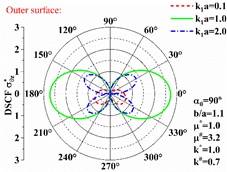Figure 3. The DSCFs of the outer wall of the circular lined tunnel in half space (Problem 2)

The undetermined constants An, Bn, Cn, Dn, En, and Fn were solved by the intercepted equation set that strictly meet the boundary conditions. The solved coefficients were substituted into the equations to test the convergence of the numerical results based on the continuity of boundaries. Dimensionless residual stresses were introduced to measure the accuracy of series solutions:

Residual stress on the outer boundary of the lined tunnel:

$\tau_{z \rho}^{b}=\left|\left.\frac{\left(\tau_{z \rho,\left(z_{2}, \bar{z}_{2}\right)}^{(S 2)}+\tau_{Z \rho,\left(z_{2}, \bar{z}_{2}\right)}^{(S 3)}+\tau_{z \rho,\left(z_{2}, \bar{z}_{2}\right)}^{(S 4)}-\tau_{z \rho,\left(z_{2}, \bar{z}_{2}\right)}^{(S T 1)}+\tau_{z \rho,\left(z_{2}, \bar{z}_{2}\right)}^{(S T 2)}\right)}{\left(i k_{1} \mu_{1} W_{0}\right)}\right|_{\left|z_{2}\right|=b}\right.$;

Residual stress on the inner boundary of the lined tunnel:

$\tau_{z \rho}^{b}=\left|\frac{\left(\tau_{z \rho,\left(z_{2}, \bar{z}_{2}\right)}^{(S T 1)}+\tau_{z \rho,\left(z_{2}, \bar{z}_{2}\right)}^{(S T 2)}\right)}{\left(i k_{1} \mu_{1} W_{0}\right)}\right|_{\left|z_{2}\right|=a}$.

Figures 4 and 5 respectively display the radial residual stresses on the inner and outer boundaries of the lined tunnel in Problem 2. It can be seen that the radial residual stresses belonged to the orders of 10-4~10-7, which are accurate enough to solve this problem.

The above verification shows that the big circle method, plus the rational interception of equation terms, can effectively solve the SH-wave scattering in half space, laying a solid basis for solving our problems.

Figures 6 and 7 respectively display the variation of $D S C F \sigma_{\theta z \max }^{*}$ with k1a on the outer wall of the partially debonded C30 concrete lining or Q345 steel lined tunnel in the sandstone layer under Condition A. In this case, the SH-wave is incident from a harder medium to a softer medium.

## 4.png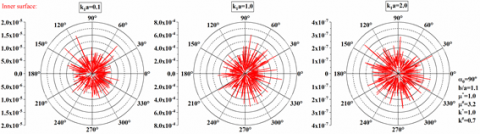Figure 4. The radial residual stresses on the inner surface in Problem 2

## 5.png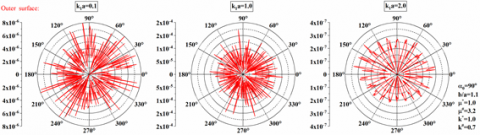Figure 5. The radial residual stresses on the outer surface in Problem 2

## 6.pngFigure 6. The variation of $D S C F \sigma_{\theta \mathrm{zmax}}^{*}$ with k1r on the outer wall of the partially debonded C30 concrete lining under Condition A

## 7.png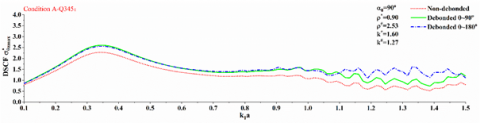Figure 7. The variation of $D S C F \sigma_{\theta z m a x}^{*}$ with k1r on the outer wall of the partially debonded Q345 steel lining under Condition A

The key of analyzing this case is to find the frequency band of concrete or steel lining that is most sensitive to the dynamic action of incident wave under Condition A. The findings will provide a theoretical basis for damping the effect of dynamic stress concentration in engineering design. Besides, the critical frequencies could be determined based on the two figures, providing a reference for subsequent discussion.

In both figures, the $D S C F \sigma_{\theta z m a x}^{*}$ of the lining increased gradually with the rise of k1a, and reached the peak at k1a≈0.3~0.4, before entering a fluctuating decline. Therefore, the lining damage induced by low-frequency wave is noteworthy, when the SH-wave is incident from a harder medium to a softer medium.

The $D S C F \sigma_{\theta \mathrm{zmax}}^{*}$ in Figure 6 appeared at k1a≈0.32, greater than the $D S C F \sigma_{\theta \mathrm{zmax}}^{*}$ in Figure 7, which was observed at k1a≈0.35. This means a high lining rigidity helps to reduce dynamic stress concentration. The k1a=0.1, 0.32, 1.25 in Figure 6 and k1a=0.1, 0.32, 1.25 in Figure 7 were taken as the critical frequencies to analyze the DSCF on the outer wall of the lined tunnel.

## 8.png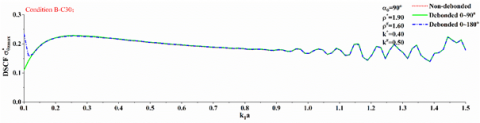Figure 8. The variation of $D S C F \sigma_{\theta \mathrm{zmax}}^{*}$ with k1a on the outer wall of the partially debonded C30 concrete lining under Condition B

## 9.pngFigure 9. The variation of $D S C F \sigma_{\theta \mathrm{zmax}}^{*}$ with k1a on the outer wall of the partially debonded Q345 steel lining under Condition B

Figures 8 and 9 respectively display the variation of $D S C F \sigma_{\theta \mathrm{zmax}}^{*}$ with k1a on the outer wall of the partially debonded C30 concrete lining or Q345 steel lined tunnel in the sandstone layer under Condition B. In this case, the SH-wave is incident from a softer medium to a harder medium.

Compared with Figures 6 and 7, the $D S C F \sigma_{\theta \mathrm{zmax}}^{*}$ values in Figures 8 and 9 were very small, for the overburden has a greater shear modulus than the lower soil layer. In both Figures 8 and 9, the $D S C F \sigma_{\theta \mathrm{zmax}}^{*}$ decreased slightly after reaching the peak at k1a≈0.25, but tended to grow after the frequency increased.

From Figures 6-9, the difference from the half space problem was summed up as: the rigidity of the overburden has a significant effect on the frequency band that corresponds to the strongest dynamic stress response of the lining. The hard overburden could shield the SH-wave. Then, k1a=0.1, 0.25, 1.25 were taken from Figures 8 and 9 as the critical frequencies to analyze the DSCF on the outer wall of the lined tunnel.

## 10.pngFigure 10. $D S C F \sigma_{\theta z}^{*}$ on the outer wall of the partially debonded C30 concrete lining in the sandstone layer under Condition A

Figure 10 provides the DSCFs on the outer wall of the partially debonded C30 concrete lining in the sandstone layer under Condition A, when the SH-wave is normally incident from the basalt layer to the sandstone layer.

It can be seen that, under a low incident frequency k1a=0.1, the $D S C F \sigma_{\theta z m a x}^{*}$ on the outer wall of the lining appeared at about 200 ° and 340 °. With the growth in incident frequency, the $D S C F \sigma_{\theta Z}^{*}$ increased significantly. Under a high incident frequency k1a=2.0, the $D S C F \sigma_{\theta Z}^{*}$ became smaller and more complex in distribution shape. In this case, the C30 concrete lining is more sensitive to the dynamic action of low-frequency incident wave, that is, the structure resonates with the site at the low frequency stage.

The debonded structure brought major changes to the distribution of DSCFs in the 0°-180° area of the lining. Debonding pushed up the $D S C F \sigma_{\theta z}^{*}$ of the outer wall of the lining in varying degrees. At k1a=0.1, the $D S C F \sigma_{\theta z}^{*}$ suddenly changed on the outer wall of the lining debonded within 0°~180°. At k1a=1.25, the $D S C F \sigma_{\theta z}^{*}$ on the outer wall of the lining increased significantly due to debonding. To sum up, debonding has varied effects on the distribution shape and value of the $D S C F \sigma_{\theta z}^{*}$ on the outer wall of the lining.

## 11.png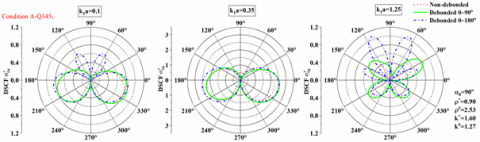Figure 11. $D S C F \sigma_{\theta z}^{*}$ on the outer wall of the partially debonded Q345 steel lining in the sandstone layer under Condition A

Figure 11 provides the DSCFs on the outer wall of the partially debonded Q345 steel lining in the sandstone layer under Condition A, when the SH-wave is normally incident from the basalt layer to the sandstone layer. Because steel has much larger density and shear modulus than concrete, the tunnel in this case has a hard and rigid lining.

Compared with Figure 10, the $D S C F \sigma_{\theta z}^{*}$ values in Figure 11 were generally small. A possible reason is that the relatively hard steel absorbs fewer energy than concrete, under dynamic actions.

Moreover, debonding exerted a greater impact on the $D S C F \sigma_{\theta z}^{*}$ on the outer wall of Q345 steel lining than that on the outer wall of C30 concrete lining: the $D S C F \sigma_{\theta z}^{*}$ on the outer wall of the steel lining was much greater than that on the outer wall of concrete lining, owing to the debonding of the lining. As shown in Figure 11, at k1a=0.1, the $D S C F \sigma_{\theta z}^{*}$ surged up in the debonded area within 0°~180°; at k1a=1.25, the $D S C F \sigma_{\theta z}^{*}$ suddenly changed on the outer wall of the debonded lining, and the dynamic stress concentration intensified with the scope of debonding.

## 12.png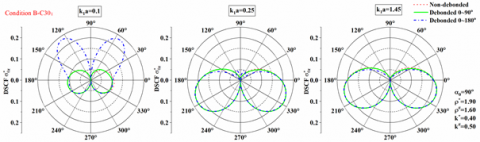Figure 12. $D S C F \sigma_{\theta z}^{*}$ on the outer wall of the partially debonded C30 concrete lining in the sandstone layer under Condition B

## 13.png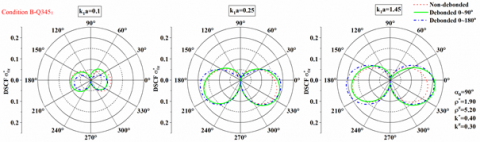Figure 13. $D S C F \sigma_{\theta z}^{*}$ on the outer wall of the partially debonded Q345 steel lining in the sandstone layer under Condition B

Figure 12 provides the DSCFs on the outer wall of the partially debonded C30 concrete lining in the sandstone layer under Condition B, when the SH-wave is normally incident from the coal seam to the sandstone layer. In this case, the SH-wave is incident from a softer medium to a harder medium.

Compared with Figure 10, the $D S C F \sigma_{\theta Z}^{*}$ values in Figure 13 were extremely small. As the shear modulus of overburden soil grew, the DSCFs around the lining in the overburden decreased across the board. This is probably the result of the fact that the hard overburden can reflect and shield the energy of the SH-wave transmitted from the lower soft soil layer, which in turn mitigates the stress concentration around the lining.

At k1a=0.1, the $D S C F \sigma_{\theta Z}^{*}$ rose sharply in the debonded area within 0°~180°. However, debonding no longer had a significant effect on DSCF, with the increase of the incident frequency.

Figure 13 provides the DSCFs on the outer wall of the partially debonded Q345 steel lining in the sandstone layer under Condition B, when the SH-wave is normally incident from the coal seam to the sandstone layer.

Similar to Figure 11, the hard overburden in Figure 13 greatly suppressed the $D S C F \sigma_{\theta Z}^{*}$ on the outer wall of the lining. Compared with Figure 12, the $D S C F \sigma_{\theta Z}^{*}$ on the outer wall of the lining was further reduced by the growing shear modulus of the lining.

In this case, debonding exerted an even greater impact on $D S C F \sigma_{\theta Z}^{*}$. In particular, when the incident frequency was k1a=0.25 or k1a=1.45, the intensity of dynamic stress concentration increased with the scope of debonding. The debonding significantly promoted the $D S C F \sigma_{\theta Z}^{*}$ on the outer wall of the lining, whether the debonding occurred within 0°~90° or 0°~180°. Therefore, the effect of SH-wave on dynamic stress concentration must be highlighted, when the steel lining debonded under condition B.

4. Conclusions

(1) The lining in soft overburden is highly sensitive to the dynamic action of low- and medium-frequency incident waves, i.e. the $D S C F \sigma_{\theta z \max }^{*}$ on the outer wall of the lining is significantly affected by low- and medium incident frequencies. The lining in hard overburden is highly sensitive to the dynamic action of high-frequency incident wave, i.e. the $D S C F \sigma_{\theta z \max }^{*}$ on the outer wall of the lining is significantly affected by high-frequency incident wave.

(2) The DSCF on the outer wall of the lining is under the joint effects of incident frequency, soil layer parameters, lining material parameters, and debonding scope. In engineering, the structure of the lined tunnel in overburn must be designed in the light of all these factors and the local geology.

(3) $D S C F \sigma_{\theta \mathrm{zmax}}^{*}$ was obviously amplified by the soft overburden, but reflected and shielded by the hard overburden. Let SMD be the shear modulus difference between the lining and surrounding soil. The smaller the SMD, the easier it is for the lining to absorb energy, and the higher the $D S C F \sigma_{\theta \mathrm{zmax}}^{*}$; the higher the SMD, the weaker the dynamic stress concentration.

(4) For the lining in soft overburden, debonding makes the lining more sensitive to the dynamic action of the low-frequency incident wave, that is, the $D S C F \sigma_{\theta \mathrm{zmax}}^{*}$ on the outer wall of the lining is affected greater at low incident frequency. For the lining in hard overburden, debonding makes the lining more sensitive to the dynamic action of the high- frequency incident wave, that is, the $D S C F \sigma_{\theta \mathrm{zmax}}^{*}$ on the outer wall of the lining is affected greater at high incident frequency. Rigid lining is affected by debonding more significantly than flexible lining.

Acknowledgment

This work was supported by the Fundamental Research Funds for Central Universities (Grant No.: 3072019CF0205).

References

 Hashash, Y.M., Hook, J.J., Schmidt, B., John, I., Yao, C. (2001). Seismic design and analysis of underground structures. Tunnelling and Underground Space Technology, 16(4): 247-293. https://doi.org/10.1016/S0886-7798(01)00051-7

 Porcu, M.C., Vielma, J.C., Panu, F., Aguilar, C., Curreli, G. (2019). Seismic retrofit of existing buildings led by non-linear dynamic analyses. International Journal of Safety and Security Engineering, 9(3): 201-212. https://doi.org/10.2495/SAFE-V9-N3-201-212

 Baron, M.L., Matthews, A.T. (1961). Diffraction of a pressure wave by a cylindrical cavity in an elastic medium. Journal of Applied Mechanics, 28(3): 205-207. https://doi.org/10.1115/1.3641710

 Mow, C.C., Mente, L.J. (1963). Dynamic stresses and displacements around cylindrical discontinuities due to plane harmonic shear waves. Journal of Applied Mechanics, 30(4): 598-604. https://doi.org/10.1115/1.3636625

 Liu, D., Gai, B., Tao, G. (1982). Applications of the method of complex functions to dynamic stress concentrations. Wave Motion, 4(3): 293-304. https://doi.org/10.1016/0165-2125(82)90025-7

 Lin, H., Liu, D.K. (2002). Scattering of SH-wave around a circular cavity in half space. Earthquake Engineering and Engineering Vibration, 22(2): 9-16. https://doi.org/10.13197/j.eeev.2002.02.002

 Davis, C.A., Lee, V.W., Bardet, J.P. (2001). Transverse response of underground cavities and pipes to incident SV waves. Earthquake Engineering & Structural Dynamics, 30(3): 383-410. https://doi.org/10.1002/eqe.14

 Liang, J.W., Zhang, H., Vincent, W.L. (2004). An analytical solution for dynamic stress concentration of underground cavities under incident plane P waves. Chinese Journal of Geotechnical Engineering, 26(6): 815-819.

 Yang, J., Qi, H. (2012). Dynamic analysis for circular inclusions of arbitrary positions near interfacial crack impacted by SH-wave in half-space. European Journal of Mechanics-A/Solids, 36: 18-24. https://doi.org/10.1016/j.euromechsol.2012.02.007

 Qi, H., Wang, Y., Liu, D.K. (2003). Dynamic analysis of shallow-embedded lining structure by incident SH-wave. Earthquake Engineering and Engineering Vibration, 23(3): 41-46. https://doi.org/10.13197/j.eeev.2003.03.007

 Coussy, O. (1984). Scattering of elastic waves by an inclusion with an interface crack. Wave Motion, 6(3): 223-236. https://doi.org/10.1016/0165-2125(84)90026-X

 Yang, Y., Norris, A.N. (1991). Shear wave scattering from a debonded fibre. Journal of the Mechanics and Physics of Solids, 39(2): 273-294. https://doi.org/10.1016/0022-5096(91)90006-A

 Norris, A., Yang, Y. (1991). Dynamic stress on a partially bonded fiber. Journal of Applied Mechanics, 58(2): 404-409. https://doi.org/10.1115/1.2897200

 Fang, X.Q., Zhang, T.F., Li, B.L., Yuan, R.J. (2020). Elastic-slip interface effect on dynamic stress around twin tunnels in soil medium subjected to blast waves. Computers and Geotechnics, 119: 103301. https://doi.org/10.1016/j.compgeo.2019.103301

 Coşkun, İ., Dolmaseven, D. (2017). Dynamic response of a circular tunnel in an elastic half space. Journal of Engineering, 2017: 6145375. https://doi.org/10.1155/2017/6145375

 Cao, H., Lee, V.W. (1989). Scattering of plane SH waves by circular cylindrical canyons with variable depth-to-width ratio. European Earthquake Engineering, 3(2): 29-37.

 Cao, H., Lee, V.W. (1979). Scattering and diffraction of plane P waves by circular cylindrical canyons with variable depth-to-width ratio. European Journal of Obstetrics & Gynecology and Reproductive Biology, 9(3): 141-150. https://doi.org/10.1016/S0301-2115(79)80003-2

 Lee, V.W., Karl, J. (1992). Diffraction of SV waves by underground, circular, cylindrical cavities. Soil Dynamics and Earthquake Engineering, 11(8): 445-456.

 Xu, H., Li, T., Xu, J., Wang, Y. (2014). Dynamic response of underground circular lining tunnels subjected to incident P waves. Mathematical Problems in Engineering, 2014: 297424. https://doi.org/10.1155/2014/297424

 Liang, J.W., Yan, L.J., Lee, V.W. (2001). Scattering of plane P waves by circular-arc layered alluvial valleys: an analytical solution. Acta Seismologica Sinica, 14(2): 176-195. https://doi.org/10.1007/s11589-001-0148-6

 Liang, J.W., Yan, L.J., Lee, V.W. (2001). Effects of a covering layer in a circular-arc canyon on incident plane SV waves. Acta Seismologica Sinica, 14(6): 660-675. https://doi.org/10.1007/BF02718077